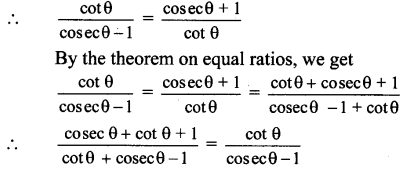# Maharashtra Board 11th Maths Solutions Chapter 2 Trigonometry – I Miscellaneous Exercise 2

Balbharti Maharashtra State Board Class 11 Maths Solutions Pdf Chapter 2 Trigonometry – I Miscellaneous Exercise 2 Questions and Answers.

## Maharashtra State Board 11th Maths Solutions Chapter 2 Trigonometry – I Miscellaneous Exercise 2

I. Select the correct option from the given alternatives.

Question 1.
The value of the expression
cos1°. cos2°. cos3° … cos 179° =
(A) -1
(B) 0
(C) $$\frac{1}{\sqrt{2}}$$
(D) 1
(B) 0

Explanation:
cos 1° cos 2° cos 3° … cos 179°
= cos 1° cos 2° cos 3° … cos 90°… cos 179°
= 0 …[∵ cos 90° = 0]Question 2.
$$\frac{\tan \mathrm{A}}{1+\sec \mathrm{A}}+\frac{1+\sec \mathrm{A}}{\tan \mathrm{A}}$$ is equal to
(A) 2cosec A
(B) 2 sec A
(C) 2 sin A
(D) 2 cos A
(A) 2cosec A

Explanation:Question 3.
If α is a root of 25cos2 θ + 5cos θ – 12 = 0, $$\frac{\pi}{2}$$ < α < π, then sin 2α is equal to
(A) $$-\frac{24}{25}$$
(B) $$-\frac{13}{18}$$
(C) $$\frac{13}{18}$$
(D) $$\frac{24}{25}$$
(A) $$-\frac{24}{25}$$

Explanation:

25 cos2 θ + 5 cos θ – 12 = 0
∴ (5cos θ + 4) (5 cos θ – 3) = 0
∴ cos θ = $$-\frac{4}{5}$$ or cos θ = $$\frac{3}{5}$$
Since $$\frac{\pi}{2}$$ < α < π,
cos α < 0
∴ cos α = $$-\frac{4}{5}$$
sin2 α = 1 – cos2 α = 1 – $$\frac{16}{25}=\frac{9}{25}$$
∴ sin α = $$\pm \frac{3}{5}$$
Since $$\frac{\pi}{2}$$ < α < π sin α > 0
∴ sin α = 3/5
sin 2 α = 2 sin α cos α
= $$2\left(\frac{3}{5}\right)\left(\frac{-4}{5}\right)=-\frac{24}{25}$$

Question 4.
If θ = 60°, then $$\frac{1+\tan ^{2} \theta}{2 \tan \theta}$$ is equal to
(A) $$\frac{\sqrt{3}}{2}$$
(B) $$\frac{2}{\sqrt{3}}$$
(C) $$\frac{1}{\sqrt{3}}$$
(D) $$\sqrt{3}$$
(B) $$\frac{2}{\sqrt{3}}$$

Explanation: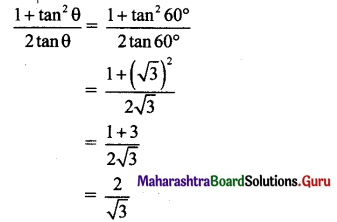Question 5.
If sec θ = m and tan θ = n, then $$\frac{1}{m}\left\{(m+n)+\frac{1}{(m+n)}\right\}$$ is equal to
(A) 2
(B) mn
(C) 2m
(D) 2n
(A) 2
Explanation: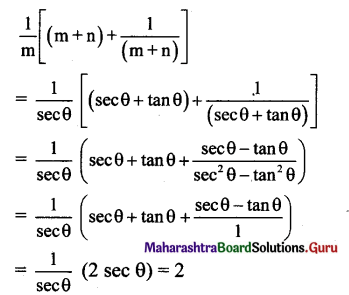Question 6.
If cosec θ + cot θ = $$\frac{5}{2}$$, then the value of tan θ is
(A) $$\frac{14}{25}$$
(B) $$\frac{20}{21}$$
(C) $$\frac{21}{20}$$
(D) $$\frac{15}{16}$$
(B) $$\frac{20}{21}$$

Explanation:
cosec θ + cot θ = $$\frac{5}{2}$$ …………….(i)
cosec2 θ – cot2 θ = 1
∴ (cosec θ + cot θ) (cosec θ – cot θ) = 1
∴ $$\frac{5}{2}$$ (cosec θ – cot θ) = 1
∴ cosec θ – cot θ = $$\frac{2}{5}$$ …(ii)
Subtracting (ii) from (i), we get
2 cot θ = $$\frac{5}{2}-\frac{2}{5}=\frac{21}{10}$$
∴ cot θ = $$\frac{21}{20}$$
∴ tan θ = $$\frac{20}{21}$$Question 7.
$$1-\frac{\sin ^{2} \theta}{1+\cos \theta}+\frac{1+\cos \theta}{\sin \theta}-\frac{\sin \theta}{1-\cos \theta}$$ equals
(A) 0
(B) 1
(C) sin θ
(D) cos θ
(D) cos θ

Explanation: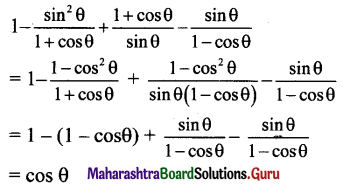Question 8.
If cosec θ – cot θ = q, then the value of cot θ is
(A) $$\frac{2 q}{1+q^{2}}$$
(B) $$\frac{2 q}{1-q^{2}}$$
(C) $$\frac{1-\mathrm{q}^{2}}{2 \mathrm{q}}$$
(D) $$\frac{1+q^{2}}{2 q}$$
(C) $$\frac{1-\mathrm{q}^{2}}{2 \mathrm{q}}$$

Explanation:

cosec θ – cot θ = q ……(i)
cosec2 θ – cot2 θ = 1
∴ (cosec θ + cot θ) (cosec θ – cot θ) = 1
∴ (cosec θ + cot θ)q = 1
∴ cosec θ + cot θ = 1/q …….(ii)
Subtracting (i) from (ii), we get
2cot θ = $$\frac{1}{\mathrm{q}}-\mathrm{q}$$
∴ cot θ = $$\frac{1-q^{2}}{2 q}$$

Question 9.
The cotangent of the angles $$\frac{\pi}{3}, \frac{\pi}{4}$$ and $$\frac{\pi}{6}$$ are in
(A) A.P.
(B) G.P.
(C) H.P.
(D) Not in progression
(B) G.P.

Explanation: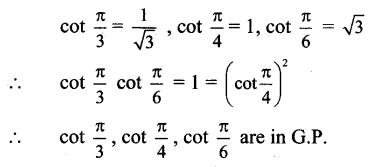Question 10.
The value of tan 1°.tan 2° tan 3° equal to
(A) -1
(B) 1
(C) $$\frac{\pi}{2}$$
(D) 2
(B) 1

Explanation:

tan1° tan2° tan3° … tan89°
= (tan 1° tan 89°) (tan 2° tan 88°)
…(tan 44° tan 46°) tan 45°
= (tan 1 ° cot 1 °) (tan 2° cot 2°)
…(tan 44° cot 44°) . tan 45°
…tan(∵ 90° – θ) = cot θ]
= 1 x 1 x 1 x … x 1 x tan 45° =1

Question 1.
Find the trigonometric functions of:
90°, 120°, 225°, 240°, 270°, 315°, -120°, -150°, -180°, -210°, -300°, -330°
Solution:
Angle of measure 90° :
Let m∠XOA = 90°
Its terminal arm (ray OA)
intersects the standard, unit circle at P(0, 1).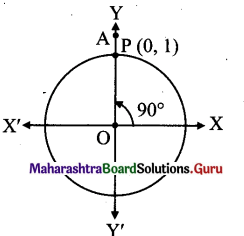∴ x = 0 and y = 1
sin 90° = y = 1
cos 90° = x = 0
tan 90° = $$\frac{y}{x}=\frac{1}{0}$$, which is not defined
cosec 90° = $$\frac{1}{y}=\frac{1}{1}$$ = 1
sec 90° = $$\frac{1}{x}=\frac{1}{0}$$, which is not defined
cot 90° = $$\frac{x}{y}=\frac{0}{1}$$ = 0

Angle of measure 120° :
Let m∠XOA =120°
Its terminal arm (ray OA) intersects the standard unit circle at P(x, y).
Draw seg PM perpendicular to the X-axis.
∴ ΔOMP is a 30° – 60° – 90° triangle.
OP = 1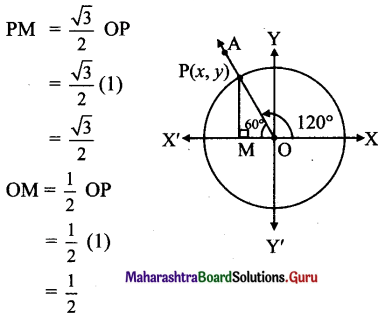Since point P lies in the 2nd quadrant, x < 0, y > 0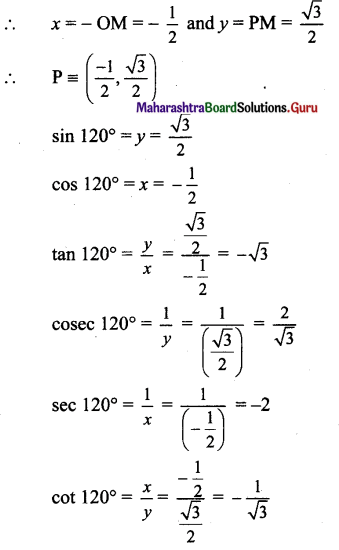[Note: Answer given in the textbook of tan 120° is $$\frac{-1}{\sqrt{3}}$$ and cot 120° is $$-\sqrt{3}$$. However, as per our $$-\sqrt{3}$$ calculation the answer of tan 120° is $$-\sqrt{3}$$ and cot 120° is $$-\frac{1}{\sqrt{3}}$$Angle of measure 225° :
Let m∠XOA = 225°
Its terminal arm (ray OA) intersects the standard unit circle at P(x, y).
Draw seg PM perpendicular to the X-axis.
ΔOMP is a 45° – 45° – 90° triangle.
OP = 1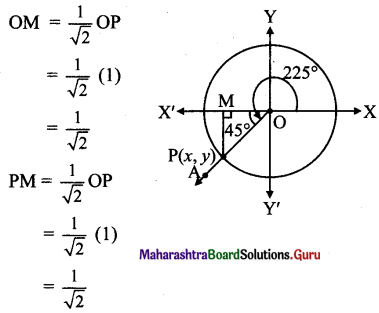Since point P lies in the 3rd quadrant, x < 0, y < 0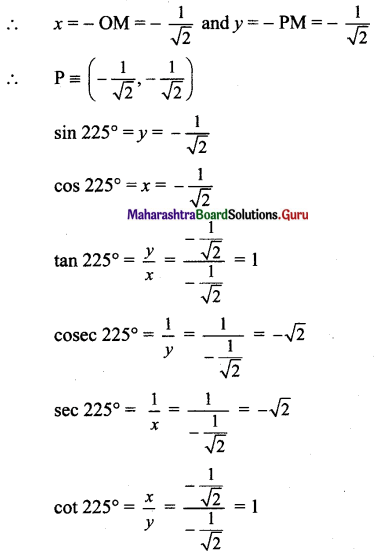Angle of measure 240° :
Let m∠XOA = 240°
Its terminal arm (ray OA) intersects the standard unit circle at P(x, y).
Draw seg PM perpendicular to the X-axis.
ΔOMP is a 30° – 60° – 90° triangle.
OP = 1Since point P lies in the 3rd quadrant, x < 0, y < 0Angle of measure 270° :
Let m∠XOA = 270°
Its terminal arm (ray OA) intersects the standard unit circle at P(0, – 1).
x = 0 andy = – 1
sin 270° = y = -1
cos 270° = x = 0
tan 270° = $$\frac{y}{x}$$Angle of measure 315° :
Let m∠XOA = 315°
Its terminal arm (ray OA) intersects the standard unit circle at P(x, y).
Draw seg PM perpendicular to the X-axis.
∴ ΔOMP is a 45° – 45° – 90° triangle.
OP = 1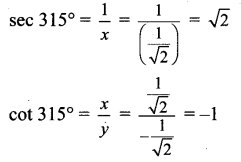[Note: Answer given in the textbook of cot 315° is 1. However, as per our calculation it is -1.]

Angle of measure (-120°):
Let m∠XOA = – 120°
Its terminal arm (ray OA) intersects the standard unit circle at P(x, y).
Draw seg PM perpendicular to the X-axis.
∴ ΔOMP is a 30° – 60° – 90° triangle.
OP = 1,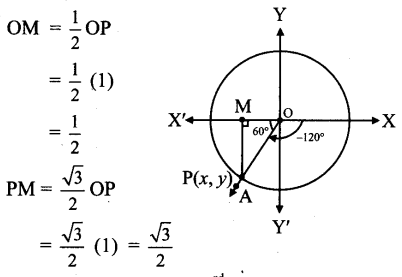Since point P lies in the 3rd quadrant, x < 0, y < 0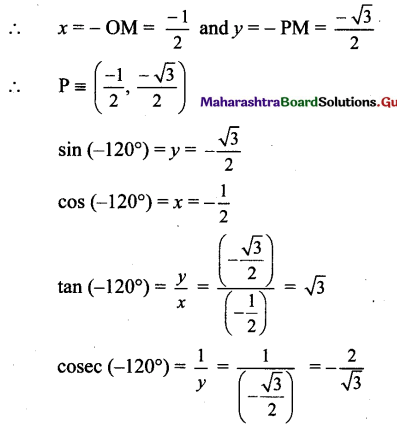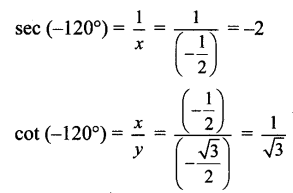Angle of measure (-150°) :
Let m∠XOA = – 150°
Its terminal arm (ray OA) intersects the standard unit circle at P(x, y).
Draw seg PM perpendicular to the X-axis.
∴ ΔOMP is a 30° – 60° – 90° triangle.
OP = 1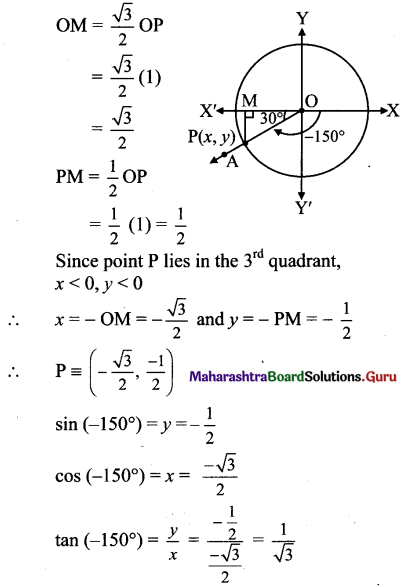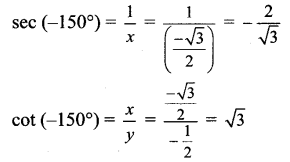Angle of measure (-180°):
Let m∠XOA = – 180°
Its terminal arm (ray OA) intersects the standard unit circle at P(- 1, 0).
∴ x = – 1 andy = 0
sin (-180°) = y = 0
cos (-180°) = x
= -1Angle of measure (- 210°):
Let m∠XOA = -210°
Its terminal arm (ray OA) intersects the standard unit circle at P(x, y).
Draw seg PM perpendicular to the X-axis.
∴ ΔOMP is a 30° – 60° – 90° triangle.
OP = 1Angle of measure (- 300°):
Let m∠XOA = – 300° Its terminal arm (ray OA) intersects the standard unit circle at P(x, y).
Draw seg PM perpendicular to the X-axis.
ΔOMP is a 30° – 60° – 90° triangle.
OP = 1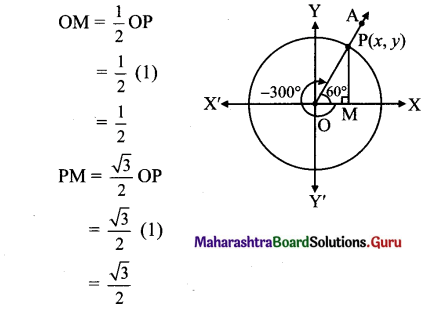Since point P lies in the 1st quadrant, x>0,y>0
x = OM = $$\frac{1}{2}$$ and
y = PM = $$\frac{\sqrt{3}}{2}$$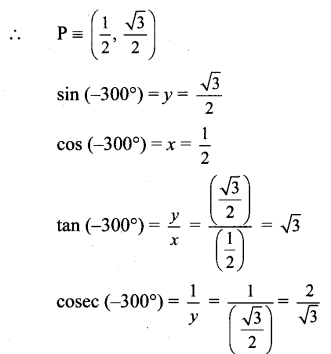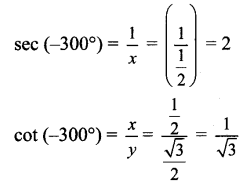Angle of measure (- 330°):
Let m∠XOA = – 330°
Its terminal arm (ray OA) intersects the standard unit circle at P(x, y).
Draw seg PM perpendicular to the X-axis.
∴ ΔOMP is a 30° – 60° – 90° triangle.
OP= 1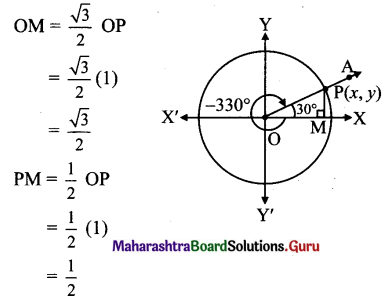Since point P lies in the 1st quadrant, x > 0, y > 0
∴ x = OM = $$\frac{\sqrt{3}}{2}$$ and y = PM = $$\frac{1}{2}$$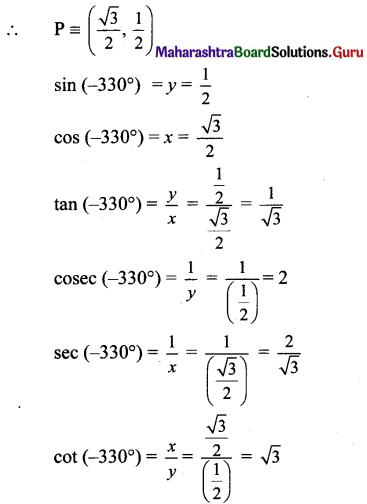Question 2.
State the signs of:
i. cosec 520°
ii. cot 1899°
iii. sin 986°
Solution:
i. 520° =360° + 160°
∴ 520° and 160° are co-terminal angles.
Since 90° < 160° < 180°,
160° lies in the 2nd quadrant.
∴ 520° lies in the 2nd quadrant,
∴ cosec 520° is positive.

ii. 1899° = 5 x 360° + 99°
∴ 1899° and 99° are co-terminal angles.
Since 90° < 99° < 180°,
99° lies in the 2nd quadrant.
∴ 1899° lies in the 2nd quadrant.
∴ cot 1899° is negative.

iii. 986° = 2x 360° + 266°
∴ 986° and 266° are co-terminal angles.
Since 180° < 266° < 270°,
266° lies in the 3rd quadrant.
∴ 986° lies in the 3rd quadrant.
∴ sin 986° is negative.Question 3.
State the quadrant in which 6 lies if
i. tan θ < 0 and sec θ > 0
ii. sin θ < 0 and cos θ < 0
iii. sin θ > 0 and tan θ < 0
Solution:
i. tan θ < 0 tan θ is negative in 2nd and 4th quadrants, sec θ > 0
sec θ is positive in 1st and 4th quadrants.
∴ θ lies in the 4th quadrant.

ii. sin θ < 0
sin θ is negative in 3rd and 4th quadrants, cos θ < 0
cos θ is negative in 2nd and 3rd quadrants.
.’. θ lies in the 3rd quadrant.

iii. sin θ > 0
sin θ is positive in 1st and 2nd quadrants, tan θ < 0
tan θ is negative in 2nd and 4th quadrants.
∴ θ lies in the 2nd quadrant.

Question 4.
Which is greater?
sin (1856°) or sin (2006°)
Solution:
1856° = 5 x 360° + 56°
∴ 1856° and 56° are co-terminal angles.
Since 0° < 56° < 90°, 56° lies in the 1st quadrant.
∴ 1856° lies in the 1st quadrant,
∴ sin 1856° >0 …(i)
2006° = 5 x 360° + 206°
∴ 2006° and 206° are co-terminal angles.
Since 180° < 206° < 270°,
206° lies in the 3rd quadrant.
∴ 2006° lies in the 3rd quadrant,
∴ sin 2006° <0 …(ii)
From (i) and (ii),
sin 1856° is greater.

Question 5.
Which of the following is positive?
sin(-310°) or sin(310°)
Solution:
Since 270° <310° <360°,
310° lies in the 4th quadrant.
∴ sin (310°) < 0
-310° = -360°+ 50°
∴ 50° and – 310° are co-terminal angles.
Since 0° < 50° < 90°, 50° lies in the 1st quadrant.
∴ – 310° lies in the 1st quadrant.
∴ sin (- 310°) > 0
∴ sin (- 310°) is positive.

Question 6.
Show that 1 – 2sin θ cos θ ≥ 0 for all θ ∈ R.
Solution:
1 – 2 sin θ cos θ
= sin2 θ + cos2 θ – 2sin θ cos θ
= (sin θ – cos θ)2 ≥ 0 for all θ ∈ R

Question 7.
Show that tan2 θ + cot2 θ ≥ 2 for all θ ∈ R.
Solution: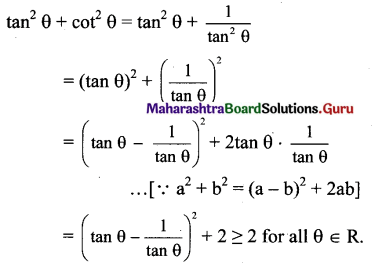Question 8.
If sin θ = $$\frac{x^{2}-y^{2}}{x^{2}+y^{2}}$$, then find the values of cos θ, tan θ in terms of x and y.
Solution:
Given, sin θ = $$\frac{x^{2}-y^{2}}{x^{2}+y^{2}}$$
we know that
cos2θ = 1 – sin2 θ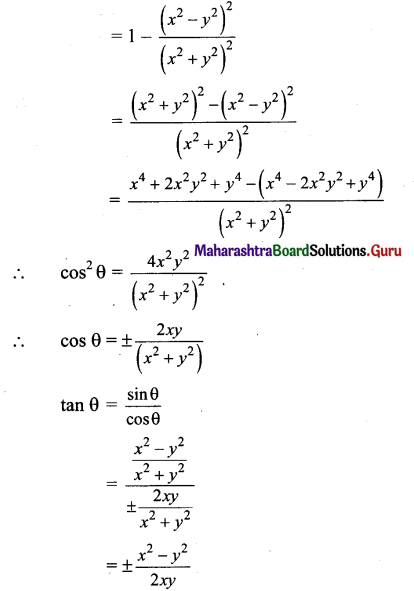[Note: Answer given in the textbook of cos θ = $$\frac{2 x y}{x^{2}+y^{2}}$$ and tan θ = $$. However, as per our calculation the answer of cos θ = ± [latex]\frac{2 x y}{x^{2}+y^{2}}$$ and tan θ = ± $$\frac{x^{2}-y^{2}}{2 x y}$$. ]Question 9.
If sec θ = $$\sqrt{2}$$ and $$\frac{3 \pi}{2}$$ < θ < 2π, then evaluate $$\frac{1+\tan \theta+{cosec} \theta}{1+\cot \theta-{cosec} \theta}$$
Solution:
Given sec θ = $$\sqrt{2}$$
We know that,
tan2 θ = sec2 θ – 1
= ($$\sqrt{2}$$) – 1
= 2 – 1 = 1
∴ tan θ = ±1
Since $$\frac{3 \pi}{2}$$ < θ < 2π
θ lies in the 4th quadrant.
∴ tan θ < 0
∴ tan θ = -1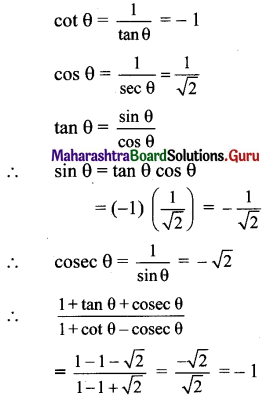Question 10.
Prove the following:

i. sin2A cos2 B + cos2A sin2B + cos2A cos2B + sin2A sin2B = 1
Solution:
L.H.S. = sin2A cos2 B + cos2A sin2B + cos2A cos2B + sin2A sin2B
= sin2A (cos2 B + sin2 B) + cos2 A (sin2 B + cos2 B)
= sin2A(1) + cos2A(1)
= 1 = R.H.S.

ii. $$\frac{(1+\cot \theta+\tan \theta)(\sin \theta-\cos \theta)}{\sec ^{3} \theta-{cosec}^{3} \theta}=\sin ^{2} \theta \cos ^{2} \theta$$
Solution: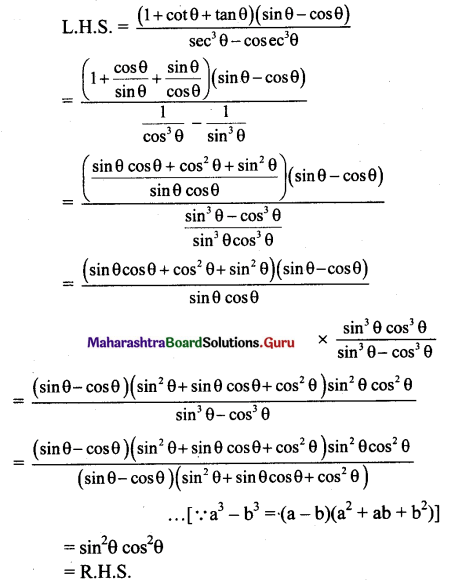iii. L.H.S. = $$\left(\tan \theta+\frac{1}{\cos \theta}\right)^{2}+\left(\tan \theta-\frac{1}{\cos \theta}\right)^{2}=2\left(\frac{1+\sin ^{2} \theta}{1-\sin ^{2} \theta}\right)$$
Solution:
L.H.S. = $$\left(\tan \theta+\frac{1}{\cos \theta}\right)^{2}+\left(\tan \theta-\frac{1}{\cos \theta}\right)^{2}$$
= (tanθ + secθ)2 + (tanθ – secθ)2
= tan2 θ + 2 tan θ sec θ + sec2 θ
+ tan2 θ – 2 tan θ sec θ +.sec2 θ
= 2(tan2 θ + sec2 θ)

iv. 2.sec2 θ – sec4 θ – 2.cosec2 θ + cosec4 θ = cot4 θ – tan4 θ
Solution:
LHS.
= 2.sec2 θ – sec4 θ – 2.cosec2 θ + cosec4 θ =  = 2 sec2 θ – (sec2 θ)2 – 2cosec2 θ + (cosec2 θ)2
= 2(1+ tan2 θ) – (1+ tan2 θ)2 – 2(1+ cot2 θ)
+ (1+ cot2 θ)2
= 2 + 2tan2 θ – (1 + 2tan2 θ + tan4 θ)
– 2 – 2cot2 θ + 1 + 2cot2 θ + cot4 θ
= 2 + 2.tan2 θ – 1 – 2 tan2 θ – tan4 θ – 2
– 2 cot2 θ + 1 + 2 cot2 θ + cot4 θ
= cot4 θ – tan4 θ = R.H.S.

v. sin4 θ + cos4 θ = sin4 θ + cos4 θ
Solution:
L.H.S. = sin4 θ + cos4 θ
= (sin2 θ)2 + (cos2 θ)2 = (sin2 θ + cos2 θ)2 – 2sin2 θ cos2 θ
… [ v a2 + b2 = (a + b)2 – 2ab]
= 1 – 2sin2 θ cos2 θ
= R.H.S.

vi. 2(sin6 θ + cos6 θ) – 3(sin4 θ + cos4 θ) + 1 = 0
L.H.S =
2(sin6 θ + cos6 θ) – 3(sin4 θ + cos4 θ) + 1=0
= sin6 θ + cos6 θ
= (sin2 θ)3 + (cos2 θ)3 = (sin2 θ + cos2 θ)3
– 3 sin2 θ cos2 θ (sin2 0 + cos2 0)
…[••• a3 + b3 = (a + b)3 – 3ab(a + b)]
= (1)3 – 3 sin2 θ cos2 θ(1)
= 1-3 sin2 θ cos2 θ sin4 θ + cos4 θ
= (sin2 θ)2 + (cos2 θ)2 = (sin2 θ + cos2 θ)2 – 2 sin2 θ cos2 θ
…[Y a2 + b2 = (a + b)2 – 2ab]
= 1-2 sin2 θ cos2 θ
L.H.S.= 2(sin6 θ + cos6 θ) – 3(sin4 θ + cos4 θ) + 1
= 2(1-3 sin2 θ cos2 θ) -3(1 – 2 sin2 θ cos2 θ) + 1
= 2-6 sin2 θ cos2 θ – 3 + 6 sin2 θ cos2 θ + 1 = c
= R.H.S.

vii. cos4 θ – sin4 θ + 1 = 2cos2θ
L.H.S. = cos4 θ – sin4 θ + 1
= (cos2 θ)2 – (sin2 θ)2 + 1 = (cos2θ + sin2θ) c(os2 θ – sin2θ) +1
= (1) (cos2θ – sin2θ) + 1 = cos2 θ + (1 – sin2θ)
= cos2 θ + cos2θ = 2cos2θ = R.H.S.

viii. sin4θ + 2sin2θ cos2θ = 1 – cos4θ
L.H.S. = sin4θ + 2sin2θ cos2θ = sin2θ(sin2θ + 2cos2θ)
= (sin2θ) (sin2θ + cos2θ + cos2θ) = (1 – cos2θ) (1 + cos2θ)
= 1 – cos4θ = R.H.S.

ix. $$\frac{\sin ^{3} \theta+\cos ^{3} \theta}{\sin \theta+\cos \theta}+\frac{\sin ^{3} \theta-\cos ^{3} \theta}{\sin \theta-\cos \theta}=2$$
Solution:= (sin2 θ + cos2 θ – sin θ cos θ) + (sin2 θ + cos2 θ + sinθ cosθ)
= 2 (sin2 θ + cos2 θ)
= 2(1)
= 2 = R.H.S.x. tan2 θ – sin2 θ = sin4 θ sec2 θ
Solution:
L.H.S. = tan2 θ – sin2 θ
= $$\frac{\sin ^{2} \theta}{\cos ^{2} \theta}$$ – sin2θ
= sin2 θ ($$\frac{1}{\cos ^{2} \theta}-1$$)
= $$\frac{\sin ^{2} \theta\left(1-\cos ^{2} \theta\right)}{\cos ^{2} \theta}$$
= (sin2 θ) (sin2 θ)sec2 θ
= sin4 θ sec2 θ
= R.H.S

xi. (sinθ + cosecθ)2 + (cos θ + see θ)2 = tan2 θ + cot2 θ + 7
Solution:
L.H.S. = (sinθ + cosecθ)2 + (cos θ + see θ)2
= sin 2 θ + cosec2 θ + 2sinθ cosec θ
+ cos2 θ + sec2 θ + 2sec0 cos0
= (sin2 θ + cos2 θ) + cosec2 θ + 2 + sec2 θ + 2
= 1 + (1 + cot2 θ) + 2 + (1 + tan2 θ) + 2 = tan2 θ + cot2 θ + 7
= R.H.S.

xii. sin8θ – cos8θ = (sin2 θ – cos2 θ) (1 – 2sin2 θ cos2 θ)
Solution:
L.H.S. = sin8θ – cos8θ
= (sin4θ)2 – (cos4θ)2
= (sin4θ – cos4θ) (sin4θ + cos4θ)
= [(sin2 θ)2 – (cos2 θ)2 ]
. [(sin2 θ)2 + (cos2 θ)2 ]
= (sin2 θ + cos2 θ) (sin2 θ – cos2 θ). [(sin2 θ + cos2 θ)2 – 2sin2 θ.cos2 θ] …[Y a2 + b2 = (a + b)2 – 2ab]
= (1) (sin2 θ – cos2 θ) (12 – 2sin2 θ cos2 θ)
= (sin2 θ – cos2 θ) (1 – 2sin2 θ cos2 θ)
= R.H.S.

xiii. sin6A + cos6A = 1 – 3 sin2A + 3sin4A
Soluiton:
L.H.S. = sin6A + cos6A
= (sin2 A)3 + (cos2 A)3
= (sin2 A + cos2 A)3
– 3sin2A cos2A(sin2 A + cos2 A)
…[ a3 + b3 = (a + b)3 – 3ab(a + b)]
= 13 – 3sin2A cos2A (1)
= 1 – 3sin2A cos2A
= 1 – 3 sin2A (1 – sin2A)
= 1 – 3 sin2A + 3sin4A
= R.H.S.

xiv. (1 + tanA tanB)2 + (tanA – tanB)2 = sec 2A sec2B
Solution:
L.H.S. = (1 + tanA tanB)2 + (tanA – tanB)2
= 1 + 2tanA tanB + tan2A tan2 + tan2 A- 2tanA tanB + tan2B
= 1 + tan2A + tan2 B + tan2A tan2B
= 1(1+ tan2A) + tan2 B(1 + tan2A)
= (1 + tan2A) (1 + tan2B)
= sec2A sec2B = R.H.S.

xv. $$\frac{1+\cot \theta+{cosec} \theta}{1-\cot \theta+{cosec} \theta}=\frac{{cosec} \theta+\cot \theta-1}{\cot \theta-{cosec} \theta+1}$$
Solution:
We know that cosec2θ – cot2 θ = 1
∴ (cosec θ – cot θ) (cosec θ + cot θ) = 1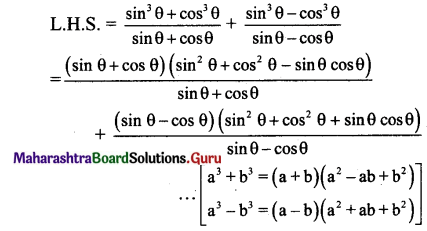xvi. $$\frac{\tan \theta+\sec \theta-1}{\tan \theta+\sec \theta+1}=\frac{\tan \theta}{\sec \theta+1}$$
Solution:
We know that
tan2θ = sec2 θ – 1
∴ tan θ. tanθ = (sec θ + 1)(sec θ – 1)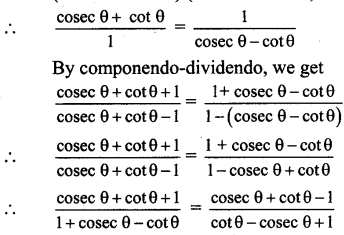xvii. $$\frac{{cosec} \theta+\cot \theta-1}{{cosec} \theta+\cot \theta+1}=\frac{1-\sin \theta}{\cos \theta}$$
Solution:
We know that,
cot2 θ = cosec2 θ – 1
∴ cot θ . cot θ = (cosec θ + 1)(cosec θ – 1)Alternate Method:xviii. $$\frac{{cosec} \theta+\cot \theta+1}{\cot \theta+{cosec} \theta-1}=\frac{\cot \theta}{{cosec} \theta-1}$$
solution:
We know that,
cot2 θ = cosec2 θ – 1
∴ cot θ.cot θ = (cosec θ + 1) (cosec θ – 1)